Ex 5.1

Chapter 5 Class 12 Continuity and Differentiability
Serial order wise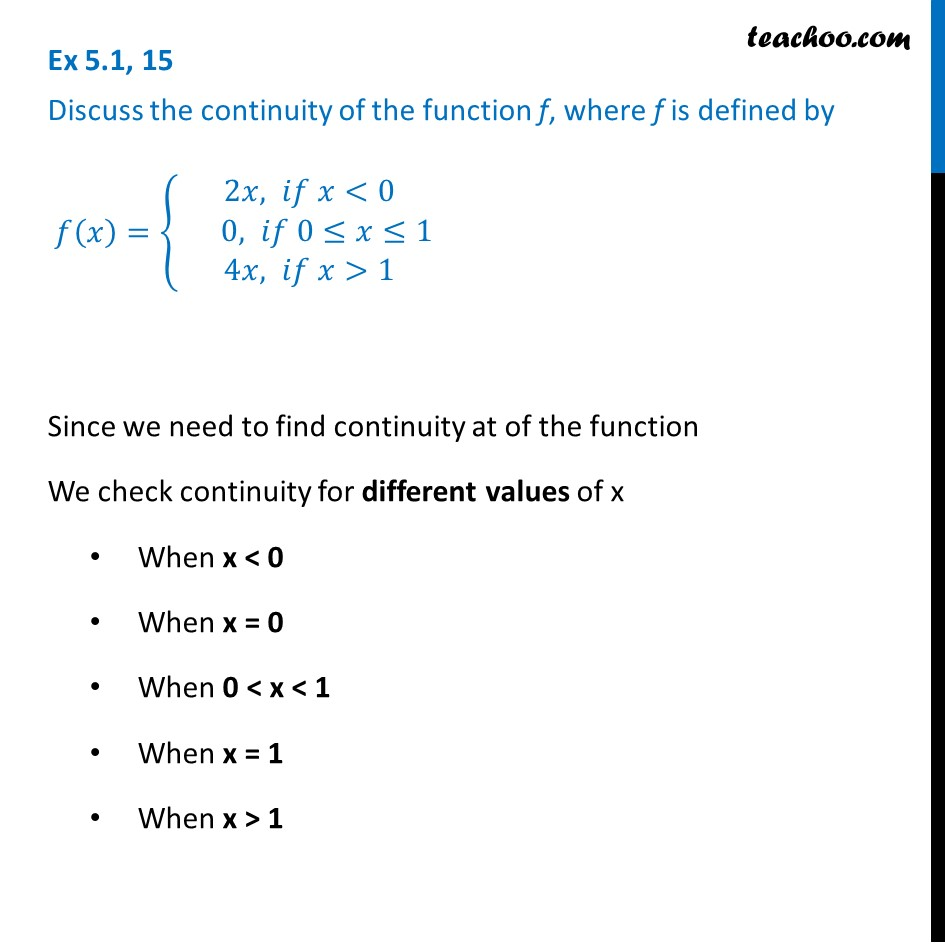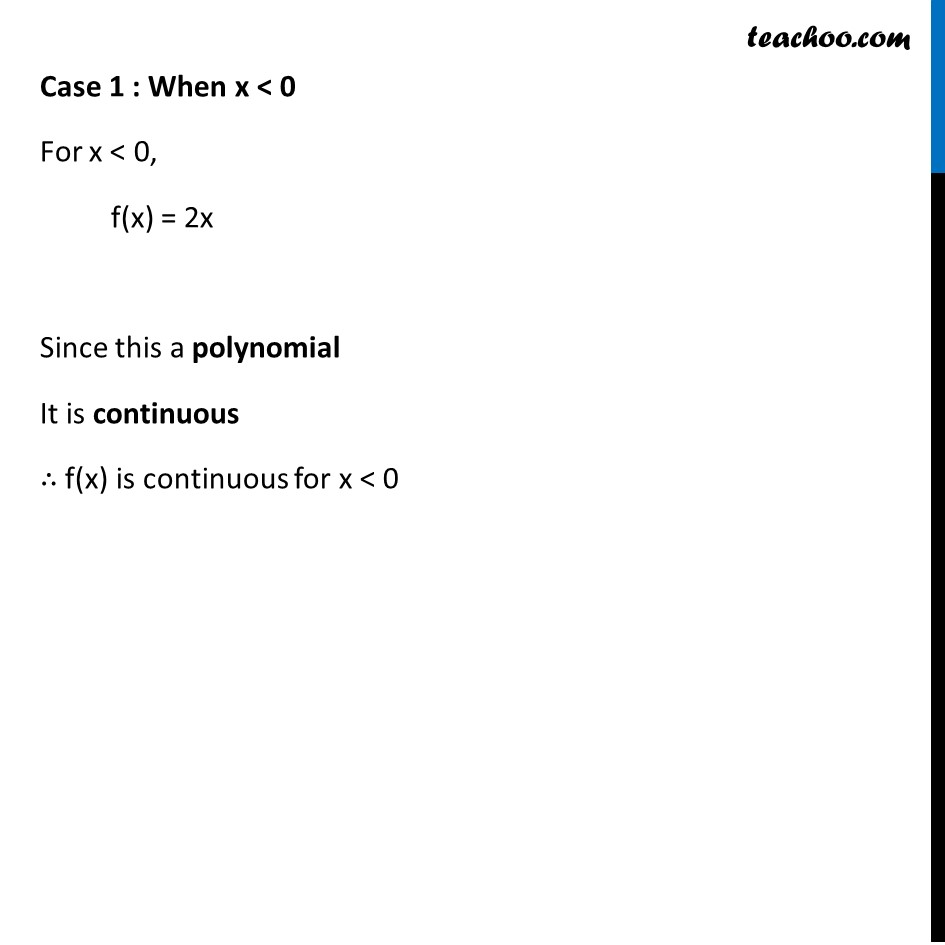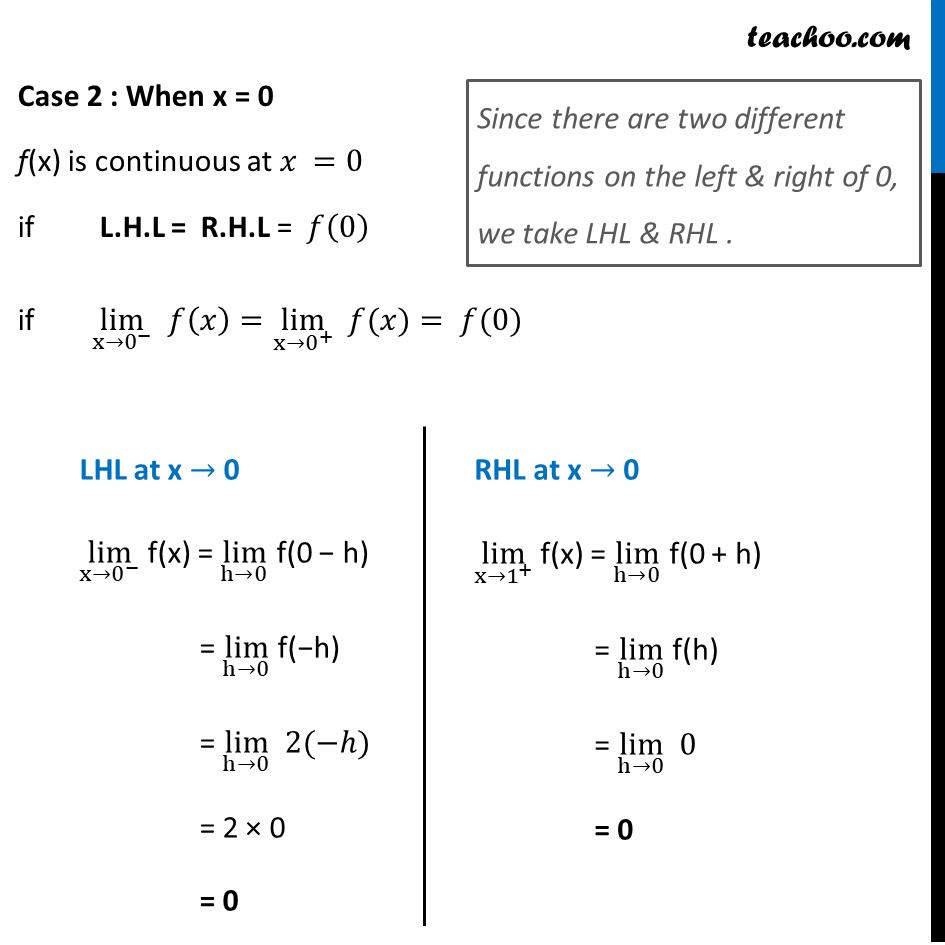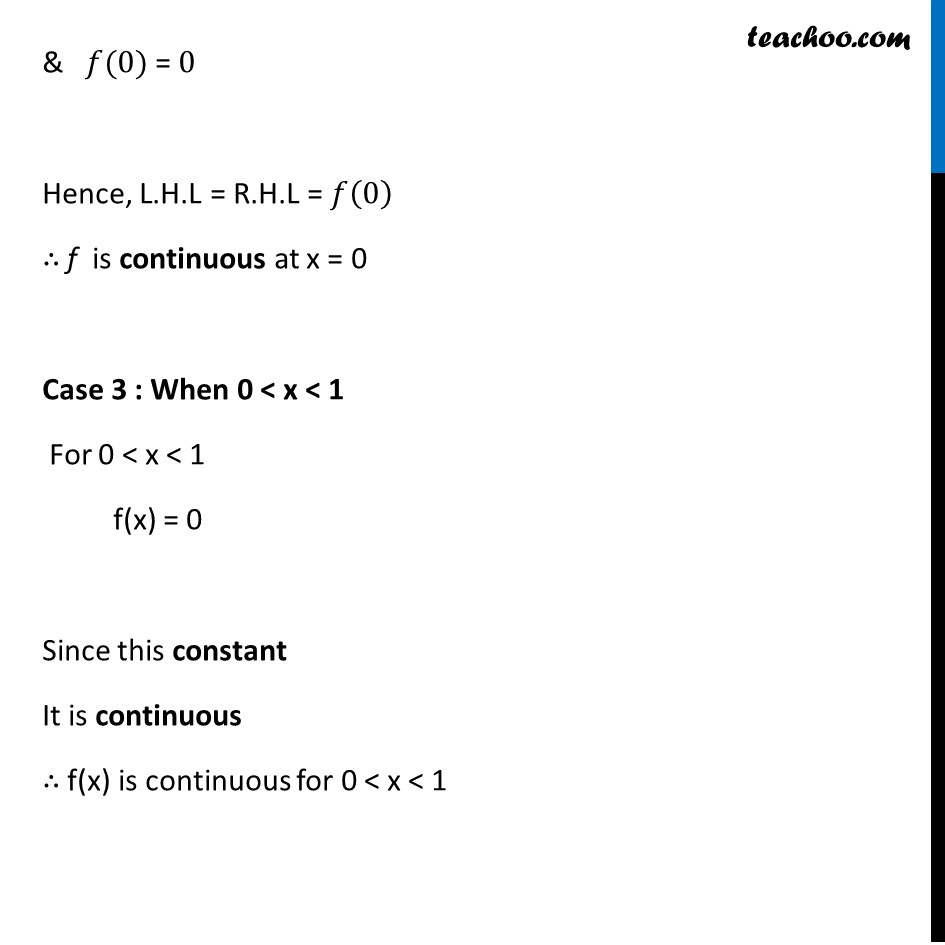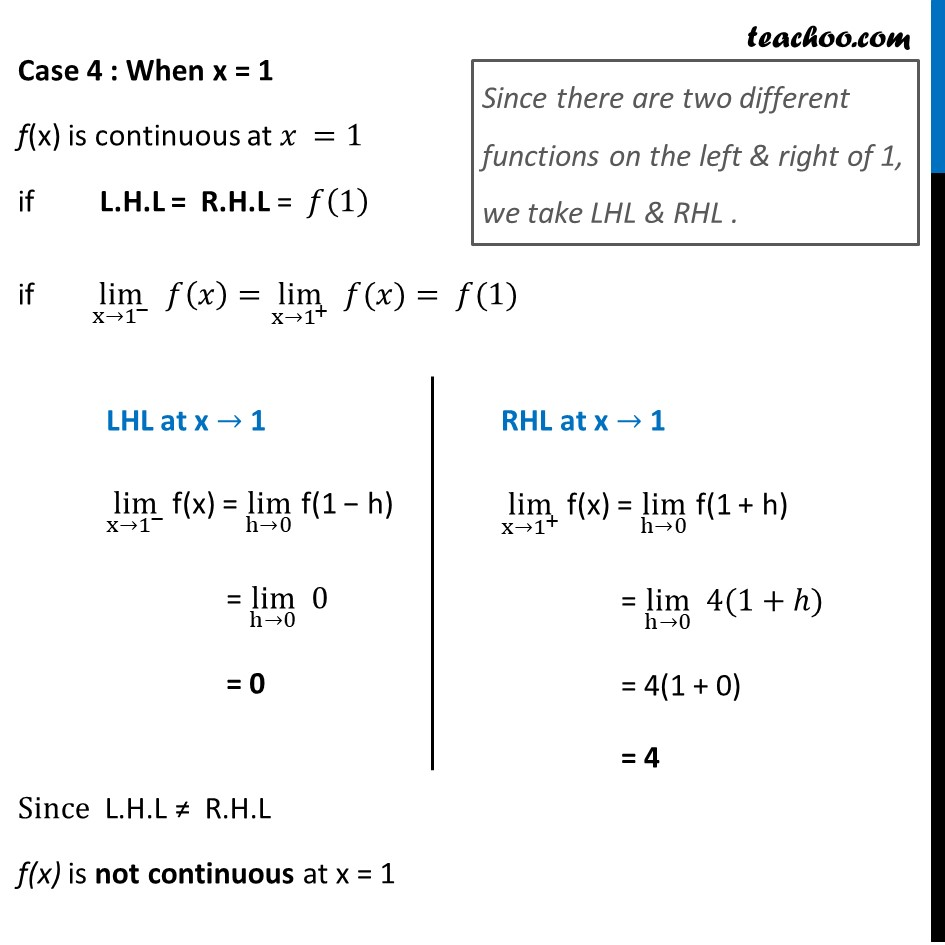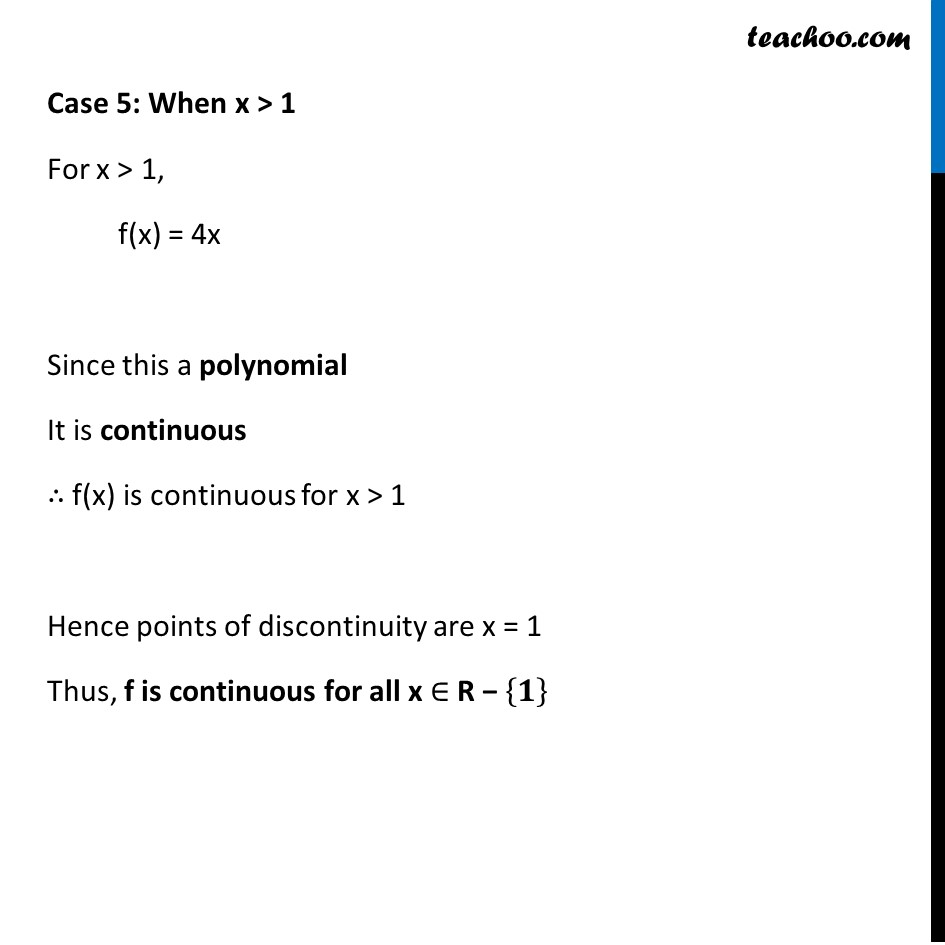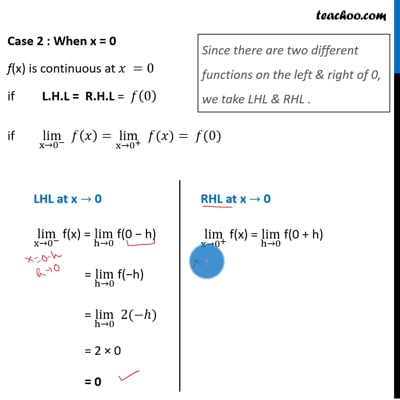This video is only available for Teachoo black users

Learn in your speed, with individual attention - Teachoo Maths 1-on-1 Class

### Transcript

Ex 5.1, 15 Discuss the continuity of the function f, where f is defined by 𝑓(𝑥)={ █(2𝑥, 𝑖𝑓 𝑥<0@ 0, 𝑖𝑓 0≤𝑥≤1@ 4𝑥, 𝑖𝑓 𝑥>1 )┤ Since we need to find continuity at of the function We check continuity for different values of x When x < 0 When x = 0 When 0 < x < 1 When x = 1 When x > 1 Case 1 : When x < 0 For x < 0, f(x) = 2x Since this a polynomial It is continuous ∴ f(x) is continuous for x < 0 Case 2 : When x = 0 f(x) is continuous at 𝑥 =0 if L.H.L = R.H.L = 𝑓(0) if lim┬(x→0^− ) 𝑓(𝑥)=lim┬(x→0^+ ) " " 𝑓(𝑥)= 𝑓(0) Since there are two different functions on the left & right of 0, we take LHL & RHL . LHL at x → 0 lim┬(x→0^− ) f(x) = lim┬(h→0) f(0 − h) = lim┬(h→0) f(−h) = lim┬(h→0) 2(−ℎ) = 2 × 0 = 0 RHL at x → 0 lim┬(x→1^+ ) f(x) = lim┬(h→0) f(0 + h) = lim┬(h→0) f(h) = lim┬(h→0) 0 = 0 & 𝑓(0) = 0 Hence, L.H.L = R.H.L = 𝑓(0) ∴ f is continuous at x = 0 Case 3 : When 0 < x < 1 For 0 < x < 1 f(x) = 0 Since this constant It is continuous ∴ f(x) is continuous for 0 < x < 1 Case 4 : When x = 1 f(x) is continuous at 𝑥 =1 if L.H.L = R.H.L = 𝑓(1) if lim┬(x→1^− ) 𝑓(𝑥)=lim┬(x→1^+ ) " " 𝑓(𝑥)= 𝑓(1) Since L.H.L ≠ R.H.L f(x) is not continuous at x = 1 Since there are two different functions on the left & right of 1, we take LHL & RHL . LHL at x → 1 lim┬(x→1^− ) f(x) = lim┬(h→0) f(1 − h) = lim┬(h→0) 0 = 0 RHL at x → 1 lim┬(x→1^+ ) f(x) = lim┬(h→0) f(1 + h) = lim┬(h→0) 4(1+ℎ) = 4(1 + 0) = 4 Case 5: When x > 1 For x > 1, f(x) = 4x Since this a polynomial It is continuous ∴ f(x) is continuous for x > 1 Hence points of discontinuity are x = 1 Thus, f is continuous for all x ∈ R − {𝟏}